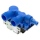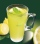# Volume - math word problems

1. Metal tubeCalculate the metal tube mass 8dm long with the outer radius 5cm and the inner radius 4.5cm and 1cm3 of this metal is 9.5g.
2. WinchThe steel rope has a diameter of 6mm and a length of 20m. We are winding on drum width 60mm, starting diameter 50mm. What is the final diameter after winding?
3. IcebergWhat is the surface area of 50 cm iceberg (in the shape of a cuboid) that can carry a man with luggage with a total weight of 120 kg?
4. Sphere floatingWill float a hollow iron ball with an outer diameter d1 = 20cm and an inside diameter d2 = 19cm in the water? The iron density is 7.8 g/cm 3. (Instructions: Calculate the average sphere density and compare with the density of the water. )
5. Triangular prismCalculate a triangular prism if it has a rectangular triangle base with a = 4cm and hypotenuse c = 50mm and height of the prism is 0.12 dm.
6. Castle modelThe castle model has a cone-shaped roof. The cone side is 45 cm long and the base radius is 27 cm. a) What is the roof volume? b) How many dm2 of wallpaper is used to glue the roof, ie the cone shell? c) What is the weight of the roof if it is made of wo
7. Pyramid heightFind the volume of a regular triangular pyramid with edge length a = 12cm and pyramid height h = 20cm.
8. Prism 4 sidesThe prism has a square base with a side length of 3 cm. The diagonal of the sidewall of the prism/BG/is 5 cm. Calculate the surface of this prism in cm square and the volume in liters
9. Cube into cylinderIf we dip a wooden cube into a barrel with a 40cm radius, the water will rise 10 cm. What is the size of the cube edge?
10. The diagram 2The diagram shows a cone with slant height 10.5cm. If the curved surface area of the cone is 115.5 cm2. Calculate correct to 3 significant figures: *Base Radius *Height *Volume of the cone
11. Water tankWhat is the height of the cuboid-shaped tank with the bottom dimensions of 80 cm and 50 cm if the 480 liters of water reaches 10 cm below the top?
12. Prism 4 sidesFind the surface area and volume four-sided prism high 10cm if its base is a rectangle measuring 8 cm and 1.2dm
13. Pile of sandA large pile of sand has been dumped into a conical pile in a warehouse. The slant height of the pile is 20 feet. The diameter of the base of the sand pile is 31 feet. Find the volume of the pile of sand.
14. The cubeThe cube has an edge of 25 cm. We cut it into small cubes of 5 cm long side. How many of these little ones left when we build a new cube of 20 cm in length?
15. The potThe pot is a cylinder with a volume of V = 7l and an inner diameter of d = 20cm. Find its depth.
16. Inlet minus outletWater flows into the tank through two pipes and flows out of the third pipe spontaneously. The pipe A itself would fill the tank in 3 hours, the pipe B itself would fille in 4 hours, and the pipe C itself would flow out in 12 hours. How long will the tank
17. Prism diagonalThe body diagonal of a regular square prism has an angle of 60 degrees with the base, the edge length is 10 cm. What is the volume of the prism?
18. Triangular pyramidWhat is the volume of a regular triangular pyramid with a side 3 cm long?
19. Pump680 liters of water were pumped in 8 minutes. How many liters was spent in 56 minutes?In January, 2 liters of lemonade were sold for CZK 24, while in February, 2.5 liters of lemonade sold at same price. How much percent was 1 liter of lemonade cheaper in February than in January?